# Assign oxidation numbers to each atom in the reaction. Note that oxidation numbers are assigned per...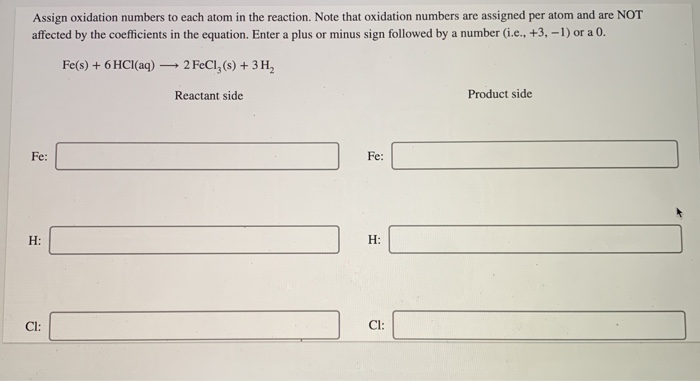Assign oxidation numbers to each atom in the reaction. Note that oxidation numbers are assigned per atom and are NOT affected by the coefficients in the equation. Enter a plus or minus sign followed by a number (i.e., +3, -1) or a 0. Fe(s) + 6HCl(aq) 2 FeCl,(s) + 3H2 Reactant side Product side Fe: Fe: H: H: CI: CI:
Show transcribed image text

This Homework Help Question: "Assign oxidation numbers to each atom in the reaction. Note that oxidation numbers are assigned per..." No answers yet.

We need 10 more requests to produce the answer to this homework help question. Share with your friends to get the answer faster!

0 /10 have requested the answer to this homework help question.

Once 10 people have made a request, the answer to this question will be available in 1-2 days.
All students who have requested the answer will be notified once they are available.
##### Add Answer of: Assign oxidation numbers to each atom in the reaction. Note that oxidation numbers are assigned per...
Similar Homework Help Questions
• ### Instructions: Assign oxidation numbers for each individual atom in all of the substances below by writing...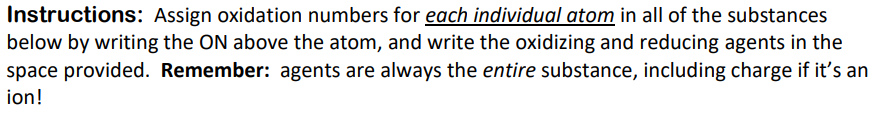Instructions: Assign oxidation numbers for each individual atom in all of the substances below by writing the ON above the atom, and write the oxidizing and reducing agents in the space provided. Remember: agents are always the entire substance, including charge if it's an ion! Fe3+(aq) + Sn2+(aq) → Fe2+(aq) + Sn4+(aq) Ox agent: Red agent: HNO2(aq) + MnO4 (aq) + Mn2+(aq) + NO3(aq) Ox agent: Red agent: SO32 (aq) + MnO4 (aq) → SO42"aq) + MnO2(aq) (not peroxide) Ox...

• ### ps Using standard rules, assign oxidation numbers to the atom reques The oxidation number of the...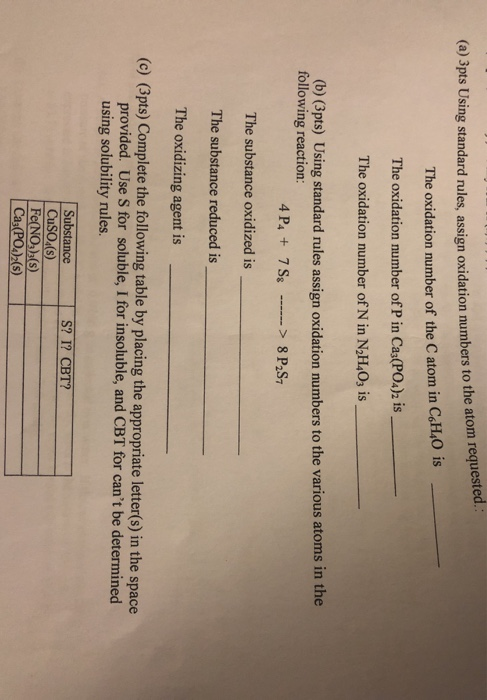ps Using standard rules, assign oxidation numbers to the atom reques The oxidation number of the Catom in C6H40 is The oxidation number of P in Ca3(PO4)2 is The oxidation number of N in N2H403 is (b) (3pts) Using standard rules assign oxidation numbers to the various atoms in the following reaction: 4P4 + 7 S8 ------> 8 P2S- The substance oxidized is The substance reduced is The oxidizing agent is (c) (3pts) Complete the following table by placing the...

• ### Be sure to answer all parts. Assign oxidation numbers to each atom in the following polyatomic...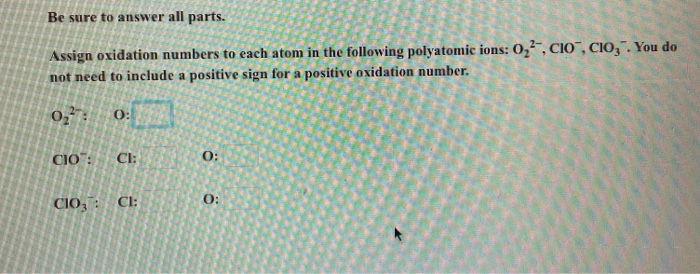Be sure to answer all parts. Assign oxidation numbers to each atom in the following polyatomic ions: 0,, not need to include a positive sign for a positive oxidation number. 02? 0: CIO": C1: 0 CIO : C1:2 0 :

• ### 2. Assign the oxidation state for all atoms in the reaction below and indicate which substance(s)...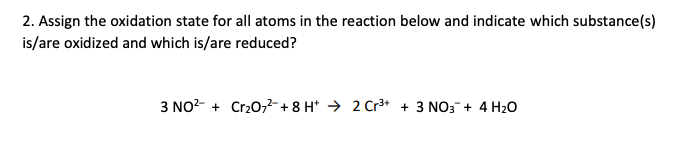2. Assign the oxidation state for all atoms in the reaction below and indicate which substance(s) is/are oxidized and which is/are reduced? 3 NO2- + Cr2O72- + 8 H → 2 Cr3+ + 3 NO3 + 4H20 Show transcribed image text

• ### Assign oxidation numbers to all of the atoms of each element in the following reaction a) Cu(s) + NO3-1(aq) => &...

Assign oxidation numbers to all of the atoms of each element in the following reaction a) Cu(s) + NO3-1(aq) =>   Cu+2(aq) + NO(g) The oxidizing agent in this reaction is_________________ The reducing agent is this reaction is_________________

• ### Іго, Electrochemistry Wolleet 1. Assign oxidation numbers to each element in the following compounds: a. VOCI...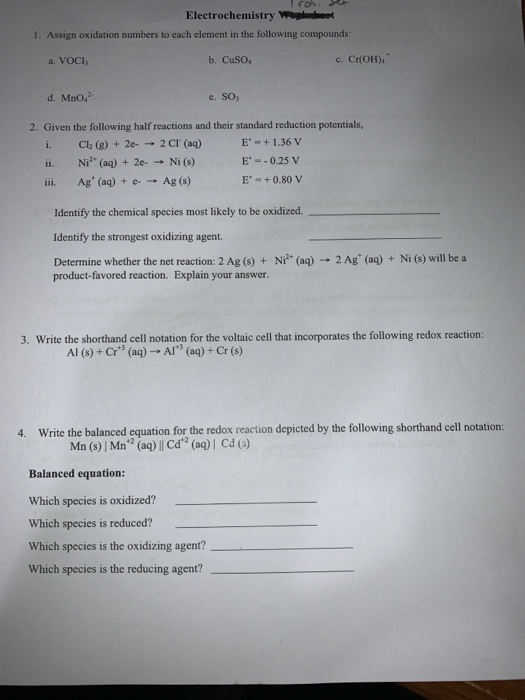Іго, Electrochemistry Wolleet 1. Assign oxidation numbers to each element in the following compounds: a. VOCI b. Cuso c. CHOH). d. Mno, e. SO, 2. Given the following half reactions and their standard reduction potentials, i. Cl (g) + 2e- - 2 CI (aq) E' =+ 1.36 V ii. Niº (aq) + 2e - Ni (s) E' = -0.25 V iii. Ag (aq) + e- Ag () E' = +0.80 V Identify the chemical species most likely to be oxidized....

• ### chem ll For the reaction KCIO, — KCI + O2 assign oxidation numbers to each element...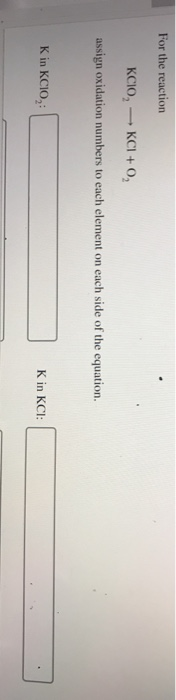chem ll For the reaction KCIO, — KCI + O2 assign oxidation numbers to each element on each side of the equation. K in KCIO: K in KCI: Question 1 of 18 > Cl in KCIO,: Clin KCI: O in KCIO, O in 0,: Which element is oxidized? Which element is reduced? OCI OK Oo ОСІ O in KCIO, in 0,: Which element is reduced? Which element is oxidized? ОСІ Ок Оо ОСІ Ок ОО In a hydrogen fuel cell,...

• ### ourseld=16056861&Open and Precipitation Reactions Part C Identify the precipitate(s) of the reaction that occurs when a...ourseld=16056861&Open and Precipitation Reactions Part C Identify the precipitate(s) of the reaction that occurs when a silver nitrate solution is mixed with a sodium chloride solution. Check all that apply. View Available Hint(s) O silver chloride O sodium chloride Pearson O silver nitrate O sodium nitrate Previous Answers ain dium chloride solution," which implies that sodium chloride is soluble does not make sense for a reactant to also be a product. ghts reserved. Terms of U 6 of 28 >...

• ### CHEM 1033, Sample Exam II (Homework) Chapters 3,4,6 Name: 1. What volume of 0.132 M KOH...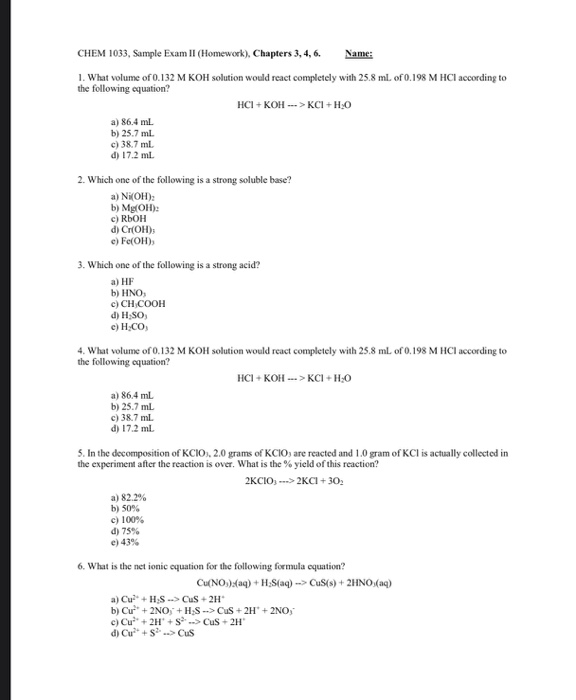CHEM 1033, Sample Exam II (Homework) Chapters 3,4,6 Name: 1. What volume of 0.132 M KOH solution would react completely with 25.8 ml. of 0.198 M HCl according to the following cquation? HCI+KOH --->KCI+H.O a) 86.4 ml. b) 25.7 ml. c) 38.7 ml d) 17.2 ml. 2. Which one of the following is a strong soluble base? a) Ni(OH): b) Mg(OH): c) RLOH d) ChOH) c) Fe(OH) 3. Which one of the following is a strong acid? a) HF b)...

Free Homework App